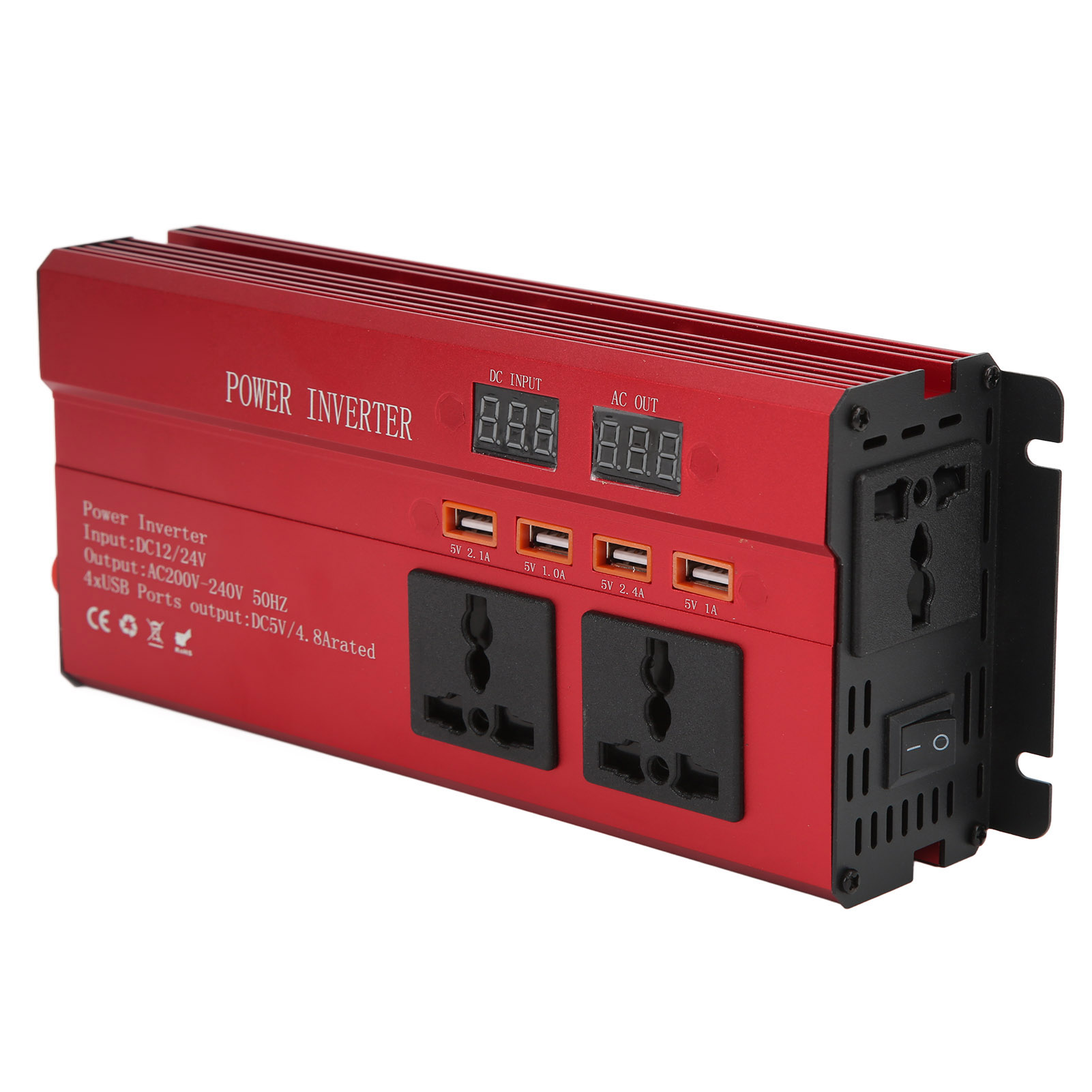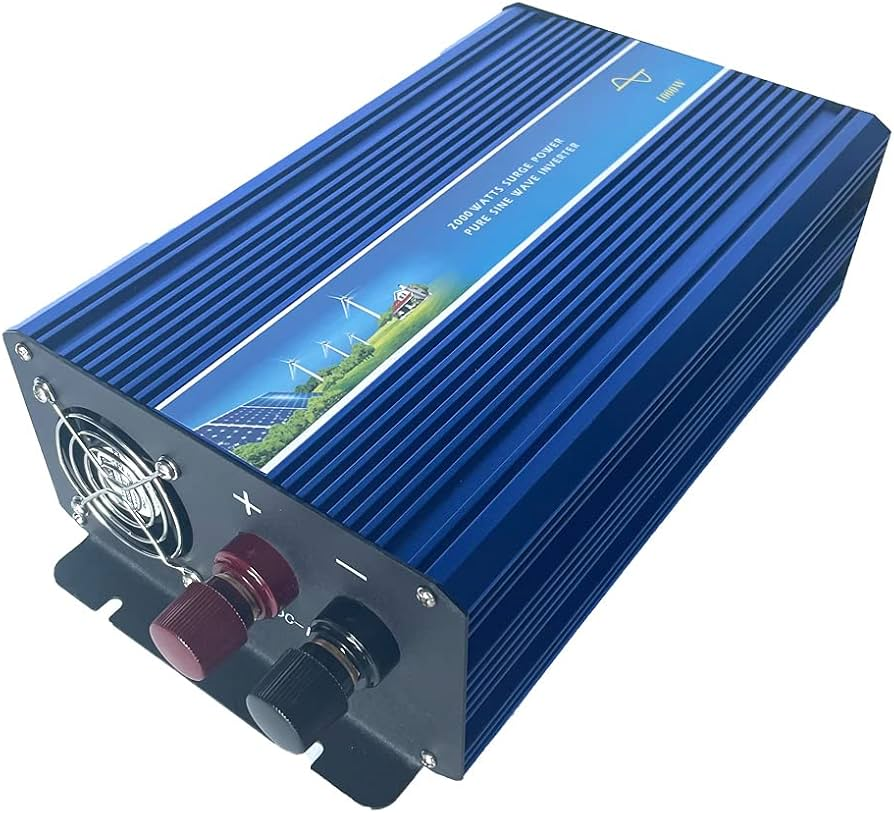# Inverter input and output voltage: What you need to know

Introduction

An inverter is a power electronic device that converts DC (direct current) electricity to AC (alternating current) electricity. Inverters are used in various applications such as solar panels, uninterruptible power supplies, and electric vehicles. Inverters are important because they allow DC-powered devices to use AC electricity, which is the standard type of electricity used in homes and businesses.

The importance of input and output voltage in inverters cannot be overstated. Input voltage refers to the voltage of the DC electricity that is being converted to AC electricity, while output voltage refers to the voltage of the resulting AC electricity. Both input and output voltage play a critical role in the performance and efficiency of an inverter.In this article, we will discuss the importance of input and output voltage in inverters, the types of input and output voltage, the relationship between input and output voltage, factors that affect input and output voltage, techniques for measuring input and output voltage, and future developments and innovations in inverter technology.

Input Voltage

Input voltage is the DC voltage that is being converted to AC voltage by an inverter. The input voltage of an inverter can come from various sources such as batteries, solar panels, or a power grid. The input voltage can be either constant or variable, depending on the source of the DC electricity.

Types of input voltage include:

1. Battery voltage – The voltage of the battery used to power the inverter. The voltage of the battery can be either 12V, 24V, or 48V, depending on the size of the battery and the application.
2. Solar panel voltage – The voltage of the solar panels that are used to power the inverter. The voltage of the solar panels can vary depending on the number of panels and the amount of sunlight.
3. Grid voltage – The voltage of the power grid that is used to power the inverter. The voltage of the power grid can vary depending on the location and time of day.

The importance of input voltage in inverters cannot be overstated. The input voltage determines the maximum power output of the inverter. If the input voltage is too low, the inverter will not be able to produce its maximum power output. If the input voltage is too high, the inverter may be damaged or even destroyed.

The ideal input voltage range for inverters depends on the design of the inverter. Most inverters have a specified input voltage range, and it is important to stay within this range to ensure optimal performance.

## III. Output Voltage

• Output voltage is the AC voltage that is produced by the inverter. The output voltage can be either constant or variable, depending on the application. The output voltage of an inverter is typically 120V or 240V, which are the standard voltages used in homes and businesses.
• Types of output voltage include:
• Pure sine wave – A pure sine wave output voltage is the most common type of output voltage produced by inverters. A pure sine wave output voltage is a smooth and consistent waveform that is similar to the waveform of the power grid.
• Modified sine wave – A modified sine wave output voltage is a waveform that has been modified from the standard sine wave waveform. Modified sine wave output voltage is cheaper to produce but can cause problems with some devices.
• The importance of output voltage in inverters cannot be overstated. The output voltage determines the type of devices that can be powered by the inverter. Some devices require a pure sine wave output voltage, while others can work with a modified sine wave output voltage.
• The ideal output voltage range for inverters depends on the application. Most inverters have a specified output voltage range, and it is important to stay within this range to ensure optimal performance.## Inverter Input and Output Voltage Relationship

• The input voltage of an inverter affects the output voltage that it produces. The output voltage of an inverter is directly proportional to the input voltage. If the input voltage increases, the output voltage will also increase, and if the input voltage decreases, the output voltage will also decrease.
• The impact of output voltage on device performance is significant. If the output voltage of an inverter is too high or too low, it can damage the device that is being powered by the inverter. Therefore, it is important to maintain the ideal input and output voltage levels for the inverter to ensure optimal performance.
• Maintaining the ideal input and output voltage levels is critical to the performance of an inverter. If the input voltage is too low, the inverter may not be able to produce its maximum power output, and if the output voltage is too high or too low, it can damage the devices being powered by the inverter.

## Factors that Affect Input and Output Voltage

There are several factors that can affect the input and output voltage of an inverter. These factors include environmental factors, inverter design, load characteristics, and maintenance issues.

Environmental factors such as temperature, humidity, and altitude can affect the performance of an inverter. High temperatures can reduce the efficiency of an inverter, and high altitudes can cause the input voltage to drop, which can affect the output voltage.Inverter design is also an important factor that affects the input and output voltage. The design of an inverter can affect its efficiency and the quality of the output voltage. A poorly designed inverter can produce a poor quality output voltage, which can affect the performance of the devices being powered by the inverter.

Load characteristics also affect the input and output voltage of an inverter. The type and size of the devices being powered by the inverter can affect the input and output voltage. Some devices require a higher input voltage, while others require a lower input voltage. The load characteristics can also affect the output voltage of the inverter.

Maintenance issues can also affect the input and output voltage of an inverter. Regular maintenance of an inverter is important to ensure optimal performance. Maintenance issues such as dirty contacts, loose connections, or worn out components can affect the input and output voltage of an inverter.

## Techniques for Measuring Inverter Input and Output Voltage

Accurate measurement of input and output voltage is important to ensure the optimal performance of an inverter. There are several common methods for measuring input voltage, such as using a multimeter or a clamp meter. A multimeter can measure the DC voltage of the battery, while a clamp meter can measure the DC voltage of the solar panels.

Techniques for measuring output voltage include using a voltmeter or an oscilloscope. A voltmeter can measure the AC voltage of the output, while an oscilloscope can provide a visual representation of the waveform of the output voltage.

Accurate measurement of input and output voltage is important to ensure optimal performance of the inverter. If the input voltage is too low or the output voltage is too high or too low, it can damage the devices being powered by the inverter.

## VII. Conclusion

In conclusion, the importance of input and output voltage in inverters cannot be overstated. The input voltage determines the maximum power output of the inverter, while the output voltage determines the type of devices that can be powered by the inverter. Maintaining the ideal input and output voltage levels is critical to the performance of an inverter.

Factors that affect input and output voltage include environmental factors, inverter design, load characteristics, and maintenance issues. Accurate measurement of input and output voltage is important to ensure optimal performance of the inverter.

Future developments and innovations in inverter technology are expected to focus on